Introduction

We consider the linear chain of N pendulums each coupled to the nearest neighbors by the elastic bonds. Let us denote the angle of rotation of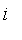-th pendulum by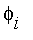and the angle velocity by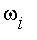. Each pendulum experiences the angular moment due to the gravity and the angular moments from the two elastic bonds, which are proportional to the difference in angles of rotation of the coupled pendulums with the coefficient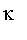. Under the assumption that all pendulums have the same moment of inertia, J, the set of equations of motion takes the form Ref.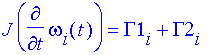where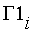is the angular moments from the elastic bonds and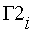is the gravity angular moment.

The angular moments from the left and right elastic bonds are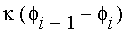and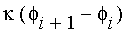, respectively and hence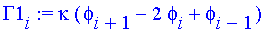The gravity angular moment can be expressed as

 > Gamma2[i]:=-M*d*g*sin(phi[i]);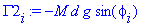where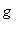is the gravity constant.

In view of the expressions for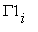and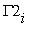, the equations of motion can be rewritten as

 > J*diff(phi[i](t),t\$2)=kappa*(phi[i+1](t)-2*phi[i](t)+phi[i-1](t))-M*d*g*sin(phi[i](t));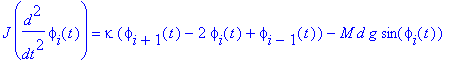The above set of equations of motion transforms in the continuous limit to the following partial differential equation

 > J*diff(phi(x,t),t\$2)=Kappa*diff(phi(x,t),x\$2)-K[G]*sin(phi(x,t));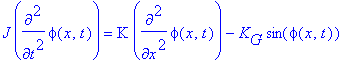where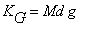, K=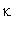h ^2.

In the new spatial and time variables,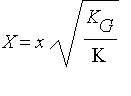,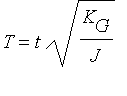, the last equation obtains the standard form of the sine-Gordon equation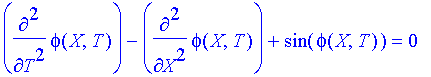One can see that the system of coupled pendulums is described by the discrete analog to the sine-Gordon equation. In the following, we will demonstrate some of the solutions to the sine-Gordon equation, using the model described above.